# Trigonometry Table

Here we will learn about the trigonometry table.

There are also trigonometry table worksheets based on Edexcel, AQA and OCR exam questions, along with further guidance on where to go next if you’re still stuck.

## What is the trigonometry table?

The trigonometry table is a table of exact values that occur for a specific angle for each of the three trigonometric functionssine, cosine and tangent.

We can use these values to calculate unknown sides, angles and areas of triangles.

The table below shows the values of trigonometric ratios of standard angles 0^{\circ}, 30^{\circ}, 45^{\circ}, 60^{\circ} and 90^{\circ}.

Step-by-step guide: Exact trig values

### What is the trigonometry table?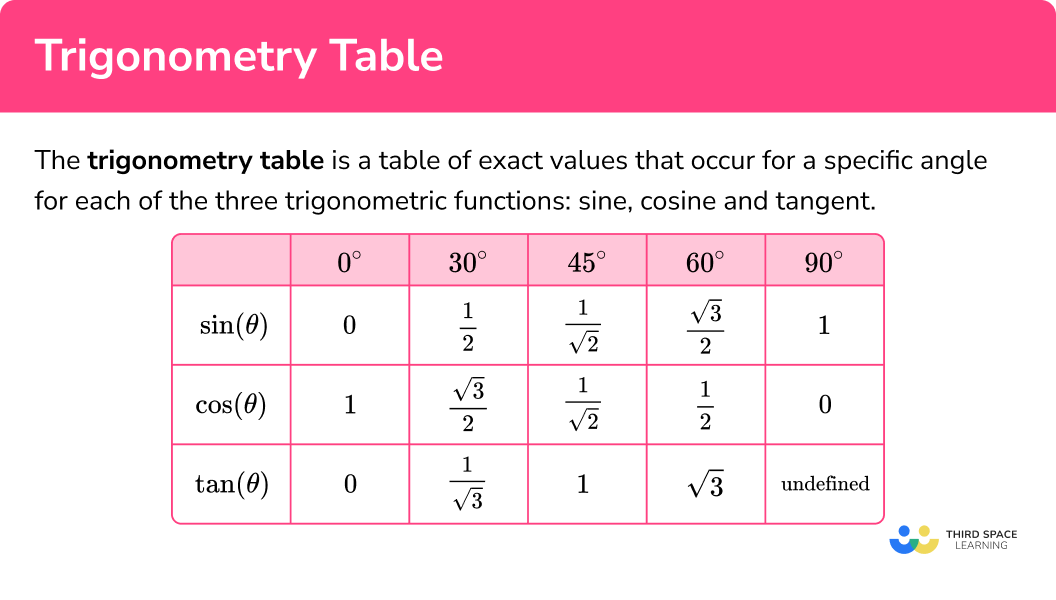### Constructing the trigonometry table

To fill in the table for the exact trig values of 30, 45 and 60 degrees, we need to construct two triangles.

• Triangle A has the interior angles of 30^{\circ}, 60^{\circ} and 90^{\circ}.
We will use triangle A to fill in the exact trig values for 30^{\circ} and 60^{\circ}.

• Triangle B has the interior angles of 45^{\circ}, 45^{\circ} and 90^{\circ}.
We will use triangle B to fill in the exact trig values for 45^{\circ}.

Using these triangles and the trig ratios sine, cosine and tangent we can complete the trigonometry table.

\sin{\theta}=\frac{O}{H} \cos{\theta}=\frac{A}{H} \tan{\theta}=\frac{O}{A}

Sine of angle \textbf{θ} is the ratio of the opposite and the hypotenuse.

Cosine function of angle \textbf{θ} is the ratio of the adjacent and the hypotenuse.

Tangent function of the angle \textbf{θ} is the ratio of the opposite and the adjacent.

### Constructing triangle A

We start with an equilateral triangle with a side length of 2 \ cm. Labelling each side as 2 \ cm and each angle as 60^{\circ}, we have,

By drawing a vertical line down the middle of the equilateral triangle, we divide it into two identical right-angled triangles.

If we look at one of these triangles, we have the following information.

Currently we do not know the height of this right-angled triangle, but we can use Pythagoras’ theorem to determine this length. With a=x, b=1 and c=2, we have,

\begin{aligned} c^{2}&=a^{2}+b^{2}\\\\ 2^{2}&=x^{2}+1^{2}\\\\ 4&=x^{2}+1\\\\ x^{2}&=4-1\\\\ x^{2}&=3\\\\ x&=\sqrt{3} \end{aligned}

Step-by-step guide: Pythagoras theorem

This means that the height of the right-angled triangle is the square root of 3 \ cm \ (\sqrt{3} \ cm). Labelling this onto the right-angled triangle, we have,

If we were to use a similar triangle, each side length would increase or decrease by the same scale factor, and the angles stay the same. This means that even if we started with an equilateral triangle that had a side length of x, we would be able to obtain exactly the same triangle as above by dividing all of the side lengths by the scale factor.

### Constructing triangle B

Now let us look at how triangle B is constructed.

We start with an isosceles right-angled triangle where the two sides with the included 90 degree angle are the same length of 1 \ cm. The two remaining angles in an isosceles triangle are equal and so they must be 45^{\circ} each.

Here, the length of the hypotenuse of the triangle is unknown but as with triangle A, we can use Pythagoras’ theorem to determine this length.

As a=1, b=1 and c=x, we have,

\begin{aligned} c^{2}&=a^{2}+b^{2}\\\\ x^{2}&=1^{2}+1^{2}\\\\ x^{2}&=1+1\\\\ x^{2}&=2\\\\ x&=\sqrt{2} \end{aligned}

So the length of the hypotenuse of triangle B is \sqrt{2} \ cm.

Any similar triangle would enlarge the side lengths, but the angles would stay the same and so any triangle containing these angles will have the same ratio of side lengths.

Step-by-step guide: Trigonometry formula

### Sin, cos and tan of 30°

Time to fill in the table of values for the three trig functions of the angles in triangle A, starting with 30^{\circ}.

Looking at the angle of 30^{\circ} we can label the sides O, A and H, accordingly.

As \sin(\theta)=\frac{O}{H}, substituting \theta=30^{\circ}, O=1 and H=2, we have \sin(30)=\frac{1}{2}.

This means that the exact trigonometric value for \sin(30) is \frac{1}{2}.

Continuing this for the cosine of 30^{\circ}, we have,

• \cos(\theta)=\frac{A}{H}, and so substituting \theta=30^{\circ}, A=\sqrt{3} and H=2, we have \cos(30)=\frac{\sqrt{3}}{2}.

This means that the exact trigonometric value for \cos(30) is \frac{\sqrt{3}}{2}.

Finally, for the tangent of 30^{\circ}, we have,

• \tan(\theta)=\frac{O}{A}, and so substituting \theta=30^{\circ}, O=1 and A=\sqrt{3}, we have \tan(30)=\frac{1}{\sqrt{3}}.

This means that the exact trigonometric value for \tan(30) is \frac{1}{\sqrt{3}}.

Filling in the trigonometry table with these values gives us,

### Sin, cos and tan of 60°

Time to fill in the table of values for the three trig functions of the angle 60^{\circ} in triangle A.

Looking at the angle of 60^{\circ} we can label the sides O, A and H, accordingly.

Notice the subtle difference that now the opposite side O=\sqrt{3} and the adjacent side A=1 where it was the other way around when we were looking at 30^{\circ}. The hypotenuse doesn’t change (H=2).

As \sin(\theta)=\frac{O}{H}, substituting \theta=60^{\circ}, O=\sqrt{3} and H=2, we have \sin(60)=\frac{\sqrt{3}}{2}.

This means that the exact trigonometric value for \sin(60) is \frac{\sqrt{3}}{2}.

Continuing this for the cosine of 60^{\circ}, we have

• \cos(\theta)=\frac{A}{H}, and so substituting \theta=60^{\circ}, A=1 and H=2, we have \cos(60)=\frac{1}{2}.

This means that the exact trigonometric value for \cos(60) is \frac{1}{2}.

Finally, for the tangent of 60^{\circ}, we have,

• \tan(\theta)=\frac{O}{A}, and so substituting \theta=60^{\circ}, O=\sqrt{3} and A=1, we have \tan(60)=\frac{\sqrt{3}}{1}=\sqrt{3}.

This means that the exact trigonometric value for \tan(60) is \sqrt{3}.

Filling in the trigonometric table with these values gives us,

Before we move on to triangle B, let’s quickly look at some common values in the table so far.

We can see that in the table above, \sin(30)=\frac{1}{2} and \cos(60)=\frac{1}{2}.

This means that \sin(30)=\cos(60).

We can also see that \sin(60)=\frac{\sqrt{3}}{2} and \cos(30)=\frac{\sqrt{3}}{2}.

This means that \sin(60)=\cos(30).

The tangent of 30^{\circ} and 60^{\circ} are similar in the sense that \tan(30)=1\div\tan(60) (they are the reciprocal of each other).

### Sin, cos and tan of 45°

Time to fill in the table of values for the three trig functions of the angle 45^{\circ} in triangle B.

Choosing one of the angles of 45^{\circ} (here we have picked the angle at the top of the triangle), we can label the sides O, A and H, accordingly.

As \sin(\theta)=\frac{O}{H}, substituting \theta=45^{\circ}, O=1 and H=\sqrt{2}, we have \sin(45)=\frac{1}{\sqrt{2}}.

This means that the exact trigonometric value for \sin(45) is \frac{1}{\sqrt{2}}.

Continuing this for the cosine of 45^{\circ}, we have

• \cos(\theta)=\frac{A}{H}, and so substituting \theta=45^{\circ}, A=1 and H=\sqrt{2}, we have \cos(45)=\frac{1}{\sqrt{2}}.

This means that the exact trigonometric value for \cos(45) is \frac{1}{\sqrt{2}}.

Finally, for the tangent of 45^{\circ}, we have

• \tan(\theta)=\frac{O}{A}, and so substituting \theta=45^{\circ}, O=1 and A=1, we have \tan(45)=\frac{1}{1}=1.

This means that the exact trigonometric value for \tan(45) is 1.

Filling in the trigonometry table with these values gives us,

Before we move on to the remaining values in the table, let us briefly inspect these new values.

We can see that in the table above, \sin(45)=\frac{1}{\sqrt{2}} and \cos(45)=\frac{1}{\sqrt{2}}.

This means that \sin(45)=\cos(45).

Also we have a pleasing value of \tan(45)=1.

To briefly summarise what we have found using the table, we have

• \sin(30)=\cos(60)=\frac{1}{2}
• \sin(60)=\cos(30)=\frac{\sqrt{3}}{2}
• \tan(60)=\frac{1}{\tan(30)}=\sqrt{3}
• \sin(45)=\cos(45)=\frac{1}{\sqrt{2}}

### Sin, cos and tan of 0°

For the sine, cosine and tangent of 0^{\circ} , we are unable to construct triangles to help us determine these values. This is because a triangle cannot contain an angle of 0^{\circ} .

Let’s look at triangle A to try and visualise what happens to the sine, cosine and tangent of the angle 30^{\circ} as it gets smaller.

As the 30^{\circ} angle at the top gets smaller, the length of the opposite side must get smaller, and the hypotenuse must get shorter as well as it tends towards the length of the adjacent side. This has different outcomes for each trigonometric function.

• As \sin(\theta)=\frac{O}{H} and the opposite side is getting smaller (tending towards 0 ), \sin(0) must also tend towards 0. This gives us the value of \sin(0)=0.
• As \cos(\theta)=\frac{A}{H} and the hypotenuse tends towards the length of the adjacent side, \cos(0) must tend towards 1 as we would divide the adjacent side by the adjacent side. This gives us the value of \cos(0)=1.
• As \tan(\theta)=\frac{O}{A} and the opposite side is getting smaller (tending towards 0 ), \tan(0) must also tend towards 0. This gives us the value of \tan(0)=0.

Filling in these three values into the first column of the table, we have,

### Sin, cos and tan of 90°

Finally we need to consider the sine, cosine and tangent of 90^{\circ}. A triangle cannot have two angles that are equal to 90^{\circ} but we can consider the sine, cosine and tangent of the angle as it increases. If we still used triangle A but this time we will refer to the angle of 60^{\circ} and increase the size of the angle towards 90^{\circ}.

As the 60^{\circ} angle at the bottom gets larger, the length of the opposite side must get longer, and the hypotenuse must get longer as well as it tends towards the length of the opposite side. This has different outcomes for each trigonometric function.

• As \sin(\theta)=\frac{O}{H} and the hypotenuse is tending towards the length of the opposite side, \sin(90) must also tend towards 1 as we would divide the opposite side by the opposite side. This gives us the value of \sin(90)=1.
• As \cos(\theta)=\frac{A}{H} and the hypotenuse is getting longer, \cos(0) must tend towards 0 as we would divide the adjacent side by a very large value for the hypotenuse, making the result incredibly small, as if it were 0. This gives us the value of \cos(90)=0.
• As \tan(\theta)=\frac{O}{A} and the opposite side is getting longer, \tan(90) is undefined as when we divide a very large number (the opposite) by a relatively small number (the adjacent), this can have little impact on the very large number. In fact, the value could be infinitely large. This means that the value of \tan(90) we use is undefined (we cannot give this a value).

Filling in these three values into the table, we have the final trigonometry table.

By looking at the table, we can now spot some other values that are the same for different trigonometric functions, including

• \sin(0)=\cos(90)=\tan(0)=0
• \sin(90)=\cos(0)=\tan(45)=1

## How to use the trigonometry table

In order to calculate using exact trig values from the trigonometry table:

1. Recall the exact trig value from the table.
2. Substitute values into the equation / expression.
3. Solve the equation / simplify the expression fully.

### Explain how to use the trigonometry table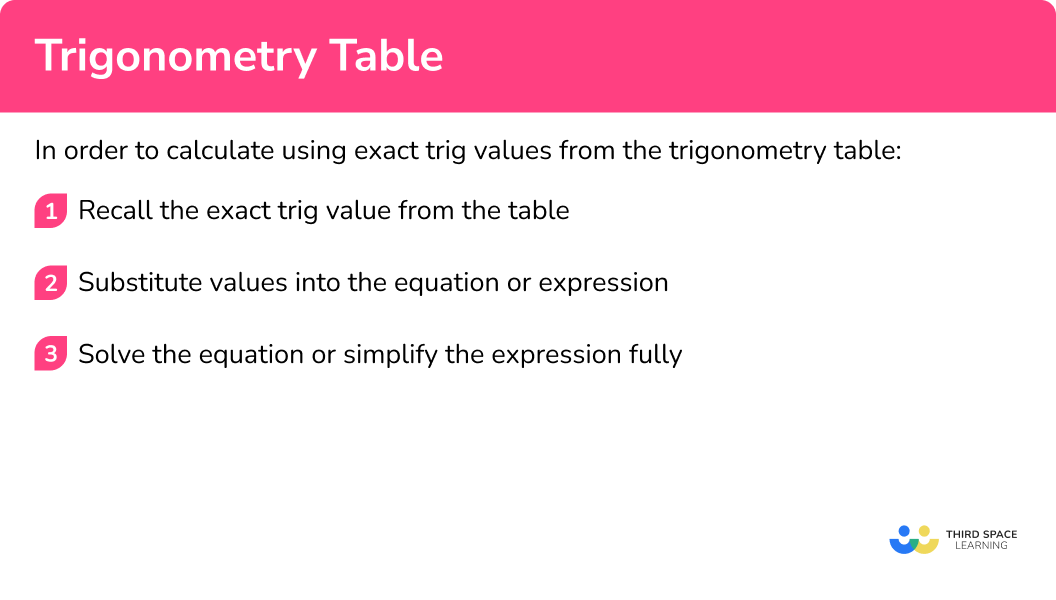## Trigonometry table examples

### Example 1: multiplying an exact trig value by an integer

Calculate the exact value of 4\cos(30).

1. Recall the exact trig value from the table.

The exact trig value of \cos(30)=\frac{\sqrt{3}}{2}.

2Substitute values into the equation / expression.

Substituting the value of \cos 30 into this expression, we have 4\cos(30)=4\times\frac{\sqrt{3}}{2}.

3Solve the equation / simplify the expression fully.

4\times\frac{\sqrt{3}}{2}=\frac{4\sqrt{3}}{2}=2\sqrt{3}

### Example 2: simplifying expressions using the trigonometry table

Simplify the expression 2\sin(45)\cos(45)-4\sin(30).

The exact trig value of \sin(45)=\frac{1}{\sqrt{2}}.

The exact trig value of \cos(45)=\frac{1}{\sqrt{2}}.

The exact trig value of \sin(30)=\frac{1}{2}.

Substituting the value of \sin{45}, the value of \cos{45} and the value of \sin{30} into the expression, we have

2\sin(45)\cos(45)-4\sin(30)=2\times\frac{1}{\sqrt{2}}\times\frac{1}{\sqrt{2}}-4\times\frac{1}{2}.

\begin{aligned} &2\times\frac{1}{\sqrt{2}}\times\frac{1}{\sqrt{2}}-4\times\frac{1}{2}\\\\ =&2\times\frac{1}{2}-2\\\\ =&1-2\\\\ =&-1 \end{aligned}

2\sin(45)\cos(45)-4\sin(30)=-1

### Example 3: dividing a surd by an exact trig value

Calculate the value of \frac{2\sqrt{2}}{\cos(45)}.

The exact trig value of \cos(45)=\frac{1}{\sqrt{2}}.

Substituting this into the expression, we have

2\sqrt{2}\div\frac{1}{\sqrt{2}}.

\begin{aligned} &2\sqrt{2}\div\frac{1}{\sqrt{2}}\\\\ =&2\sqrt{2}\times\sqrt{2}\\\\ =&2\times\sqrt{4}\\\\ =&2\times{2}\\\\ =&4 \end{aligned}

The value of \frac{2\sqrt{2}}{\cos(45)}=4.

### Example 4: calculating with three exact trig values

Calculate the exact value of \sin(30)+\cos(60)-\tan(45).

The exact trig value of \sin(30)=\frac{1}{2}.

The exact trig value of \cos(60)=\frac{1}{2}.

The exact trig value of \tan(45)=1.

Substituting these into the expression, we have

\sin(30+\cos(60)-\tan(45)=\frac{1}{2}+\frac{1}{2}-1.

\frac{1}{2}+\frac{1}{2}-1=1-1=0

\sin(30)+\cos(60)-\tan(45)=0

### Example 5: solving equations with exact trig values

Given that x=\cos(30), solve the equation 3y=(2x)^{2}.

Using the trigonometry ratios table, the exact trig value of \cos(30)=\frac{\sqrt{3}}{2}.

Substituting this into the equation 3y=(2x)^{2}, we have

3y=(2\times\frac{\sqrt{3}}{2})^{2}.

\begin{aligned} 3y&=(2\times\frac{\sqrt{3}}{2})^{2}\\\\ 3y&=(\sqrt{3})^{2}\\\\ 3y&=3\\\\ y&=1 \end{aligned}

### Example 6: solving equations with exact trig values

Given that x=\sin(45) and y=\cos(45), determine the value of \theta of the equation \tan(\theta)=\frac{x}{y}.

The exact trig value of \sin(45)=\frac{1}{\sqrt{2}}.

The exact trig value of \cos(45)=\frac{1}{\sqrt{2}}.

Substituting these values into the equation, we have

\tan(\theta)=\frac{1}{\sqrt{2}}\div\frac{1}{\sqrt{2}}.

\frac{1}{\sqrt{2}}\div\frac{1}{\sqrt{2}}=1 and so we now have the equation \tan(\theta)=1.

As \tan(45)=1 from the trig table, we can state that

\theta=45^{\circ}.

Note that algebraically we would write the inverse \tan of 1 is 45 \ (\tan^{-1}(1)=45^{\circ}).

### Common misconceptions

• Exact values

There is no need to write your answer as a decimal and round. It would no longer be an exact value.

• Using exact values

If you have been given the answer, you need to make it clear that you have used the exact trig value in your workings.

### Practice trigonometry table questions

1. What is the value of \theta when \cos(\theta)=1?

0^{\circ}30^{\circ}45^{\circ}90^{\circ}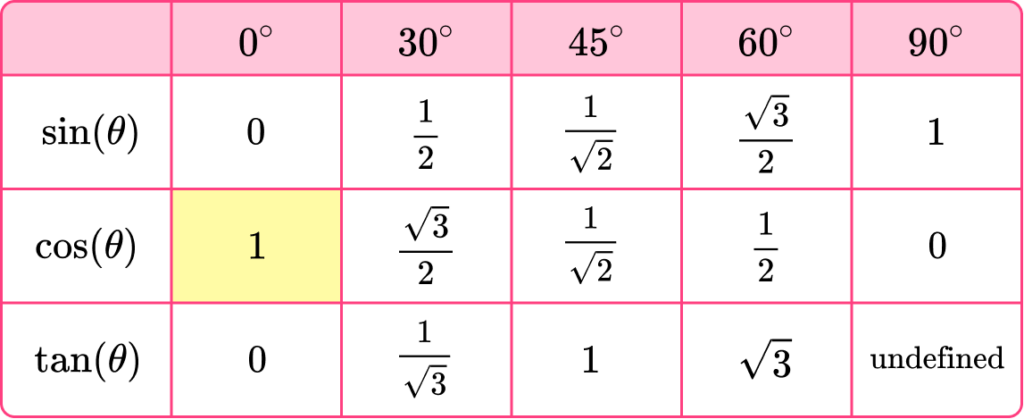2. Simplify the expression 2\sin(30)+3\tan(45).

3+\sqrt{3}43+\frac{2}{\sqrt{3}}1952\sin(30)=2\times\frac{1}{2}=1.

3\tan(45)=3\times{1}=3.

1+3=4.

3. Calculate the value of \frac{\cos(30)}{\sqrt{3}}.

\frac{1}{3}\frac{1}{2}0.95\text{ (2dp)}3\cos(30)=\frac{\sqrt{3}}{2}

\frac{\sqrt{3}}{2}\div\sqrt{3}=\frac{\sqrt{3}}{2}\times\frac{1}{\sqrt{3}}=\frac{1}{2}

4. Calculate the exact value of 2(\cos(30)+\sin(60))-\tan(0).

21+\sqrt{3}2\sqrt{3}1802(\cos(30)+\sin(60))-\tan(0)=2(\frac{\sqrt{3}}{2}+\frac{\sqrt{3}}{2})-0=2\sqrt{3}

5. Given that x=\tan(30), solve the equation 2y=(6x)^{2}-2.

534106102y=(6\tan(30))^{2}-2

2y=(6\times\frac{1}{\sqrt{3}})^{2}-2

2y=\frac{36}{3}-2

2y=12-2=10

Therefore y=5 .

6. Given that x=\sin(30) and y=\cos(60), determine the value of \theta of the equation \sin(\theta)=4xy.

0^{\circ}45^{\circ}60^{\circ}90^{\circ}\sin(\theta)=4\sin(30)\cos(60)=4\times\frac{1}{2}\times\frac{1}{2}=1

\sin(90)=1 and so \theta=90^{\circ}.

### Trigonometry table GCSE questions

1. Complete the table of exact trigonometric values for the angles of 30^{\circ} and 60^{\circ}.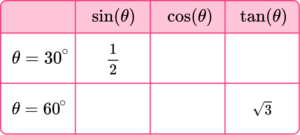(4 marks)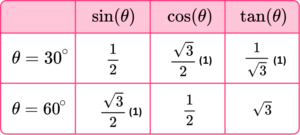(4)

2. James sketches the right-angled triangle used to generate the exact trig values for the sine, cosine and tangent of 45^{\circ}. His sketch is drawn below.

(a) State the mistake that James has made with his sketch.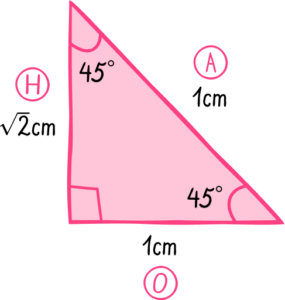(b) Calculate the actual area of the triangle that James was meant to sketch.

(3 marks)

(a)

Any of the following.

He has mislabelled the hypotenuse as the perpendicular height.

The perpendicular height is longer than the hypotenuse (\sqrt{2} and 1).

The adjacent side should be the perpendicular height.

The hypotenuse should be the side opposite the right angle.

The two perpendicular sides should be the same length.

The side adjacent to the 45^{\circ} angle at the top is the perpendicular height.

(1)

(b)

1\times{1}\div{2}

(1)

0.5 \ cm^{2}

(1)

3. Triangles ABE and ACD are similar.

• AB = 1 \ cm
• BE = 0.5 \ cm
• CD = 1 \ cm
• Angle ABE = 60^{\circ}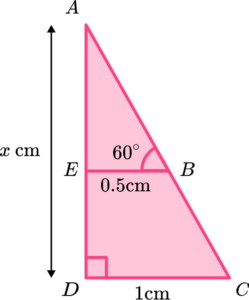Determine the length of the line AE = x \ cm.

(5 marks)

\tan(60)=\sqrt{3}

(1)

AE=0.5\times \sqrt{3}=\frac{\sqrt{3}}{2}

(1)

Scale factor is 1\div 0.5=2 .

(1)

(1)

x=\sqrt{3}

(1)

## Learning checklist

You have now learned how to:

• Know the exact values of \sin \theta and \cos \theta for \theta = 0^{\circ}, 30^{\circ}, 45^{\circ}, 60^{\circ} and 90^{\circ} ; know the exact value of \tan \theta for \theta = 0^{\circ}, 30^{\circ}, 45^{\circ}, 60^{\circ}

## Beyond GCSE

Other trigonometric functions covered at A level include,

• Secant
The secant function (sec) is 1 divided by the cosine of an angle.

• Cosecant
The cosecant function (cosec) is 1 divided by the sine of an angle.

• Cotangent
The cotangent function (cot) is 1 divided by the tangent of an angle.

We also use a different unit of measure for angles called radians.

## Still stuck?

Prepare your KS4 students for maths GCSEs success with Third Space Learning. Weekly online one to one GCSE maths revision lessons delivered by expert maths tutors.

Find out more about our GCSE maths tuition programme.